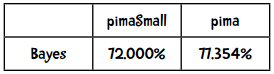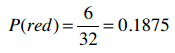## 使用Python实现

### 训练阶段

P(共和党) = 233 / 433 = 0.54

``````{'democrat': {'bill 1': {'yes': 0.333, 'no': 0.667},
'bill 2': {'yes': 0.778, 'moderate': 0.222}},
'republican': {'bill 1': {'yes': 0.811, 'no': 0.189},
'bill 2': {'yes': 0.250, 'no': 0.750}}}
``````

``````mean = {'democrat': {'age': 57, 'years served': 12},
'republican': {'age': 53, 'years served': 7}}
ssd = {'democrat': {'age': 7, 'years served': 3},
'republican': {'age': 5, 'years served': 5}}
``````• `attr`表示这一列是分类型的特征
• `num` 表示这一列是数值型的特征
• `class` 表示这一列是分类

``````"num    num    num    num    num    num    num    num    class"
````````````totals = {'1': {1: 8, 2: 378, 3: 182, 4: 102, 5: 1141,
6: 98.2, 7: 2.036, 8: 141},
'0': {1: 3, 2: 323, 3: 242, 4: 96, 5: 214,
6: 98.1, 7: 2.006, 8: 76}}
``````

``````numericValues = {'1': {1: [3, 4, 1], 2: [78, 111, 189], ...},
'0': {1: [2, 1, 0], 2: [142, 81, 100], ...}}
``````

``````import math

class Classifier:
def __init__(self, bucketPrefix, testBucketNumber, dataFormat):
"""bucketPrefix 分桶数据集文件前缀
testBucketNumber 测试桶编号
dataFormat 数据格式，形如：attr attr attr attr class
"""
total = 0
classes = {}
# 对分类型数据进行计数
counts = {}
# 对数值型数据进行求和
# 我们会使用下面两个变量来计算每个分类各个特征的平均值和样本标准差
totals = {}
numericValues = {}

# 从文件中读取数据
self.format = dataFormat.strip().split('\t')
self.prior = {}
self.conditional = {}

# 遍历1-10号桶
for i in range(1, 11):
# 判断是否跳过
if i != testBucketNumber:
filename = "%s-%02i" % (bucketPrefix, i)
f = open(filename)
f.close()
for line in lines:
fields = line.strip().split('\t')
ignore = []
vector = []
nums = []
for i in range(len(fields)):
if self.format[i] == 'num':
nums.append(float(fields[i]))
elif self.format[i] == 'attr':
vector.append(fields[i])
elif self.format[i] == 'comment':
ignore.append(fields[i])
elif self.format[i] == 'class':
category = fields[i]
# 处理这条记录
total += 1
classes.setdefault(category, 0)
counts.setdefault(category, {})
totals.setdefault(category, {})
numericValues.setdefault(category, {})
classes[category] += 1
# 处理分类型数据
col = 0
for columnValue in vector:
col += 1
counts[category].setdefault(col, {})
counts[category][col].setdefault(columnValue, 0)
counts[category][col][columnValue] += 1
# 处理数值型数据
col = 0
for columnValue in nums:
col += 1
totals[category].setdefault(col, 0)
#totals[category][col].setdefault(columnValue, 0)
totals[category][col] += columnValue
numericValues[category].setdefault(col, [])
numericValues[category][col].append(columnValue)

# 计算先验概率P(h)
for (category, count) in classes.items():
self.prior[category] = count / total

# 计算条件概率P(h|D)
for (category, columns) in counts.items():
self.conditional.setdefault(category, {})
for (col, valueCounts) in columns.items():
self.conditional[category].setdefault(col, {})
for (attrValue, count) in valueCounts.items():
self.conditional[category][col][attrValue] = (
count / classes[category])
self.tmp =  counts

# 计算平均值和样本标准差
self.means = {}
self.ssd = {}
# 动手实践
``````

``````>>> c = Classifier('pimaSmall/pimaSmall', 1, 'num\tnum\tnum\tnum\tnum\tnum\tnum\tnum\tclass')
>>> c.ssd
{'1': {1: 4.21137914295475, 2: 29.52281872377408, ...},
'0': {1: 2.54694671925252, 2: 23.454755259159146, ...}}
>>> c.means
{'1': {1: 5.25, 2: 146.05555555555554, ...},
'0': {1: 2.8867924528301887, 2: 111.90566037735849, ...}}
``````

``````        # 计算平均值和样本标准差
self.means = {}
self.ssd = {}

for (category, columns) in totals.items():
self.means.setdefault(category, {})
for (col, cTotal) in columns.items():
self.means[category][col] = cTotal / classes[category]

for (category, columns) in numericValues.items():
self.ssd.setdefault(category, {})
for (col, values) in columns.items():
SumOfSquareDifferences = 0
theMean = self.means[category][col]
for value in values:
SumOfSquareDifferences += (value - theMean)**2
columns[col] = 0
self.ssd[category][col] = math.sqrt(SumOfSquareDifferences / (classes[category]  - 1))
````````````    def classify(self, itemVector, numVector):
"""返回itemVector所属分类"""
results = []
sqrt2pi = math.sqrt(2 * math.pi)
for (category, prior) in self.prior.items():
prob = prior
col = 1
for attrValue in itemVector:
if not attrValue in self.conditional[category][col]:
# 该特征值没有出现过，因此概率给0
prob = 0
else:
prob = prob * self.conditional[category][col][attrValue]
col += 1
col = 1
for x in  numVector:
mean = self.means[category][col]
ssd = self.ssd[category][col]
ePart = math.pow(math.e, -(x - mean)**2/(2*ssd**2))
prob = prob * ((1.0 / (sqrt2pi*ssd)) * ePart)
col += 1
results.append((prob, category))
# 返回概率最高的分类
#print(results)
return(max(results))
``````

### 朴素贝叶斯的效果要比近邻算法好吗？kNN算法中，k=3时的Kappa指标是0.35415，效果一般。那朴素贝叶斯的Kappa指标是多少呢？• 实现简单（只需计数即可）
• 需要的训练集较少
• 运算效率高

kNN算法的优点：

• 实现也比较简单
• 不需要按特定形式准备数据
• 需要大量内存保存训练集数据• 运动员例子中，身高和体重不是互相独立的，因为高的人体重也会较高。
• 地区邮编、收入、年龄，这些特征也不完全独立，一些地区的房屋都很昂贵，一些地区则只有房车：加州帕罗奥图大多是20岁的年轻人，而亚利桑那州则多是退休人员。
• 在音乐基因工程中，很多特征也是不独立的，如果音乐中有很多变音吉他，那小提琴的概率就降低了。
• 血液检验的结果中，T4和TSH这两个指标通常是呈反比的。

``````>>> tenfold('mpgData/mpgData', 'class\tattr\tnum\tnum\tnum\tnum\tcomment')
``````